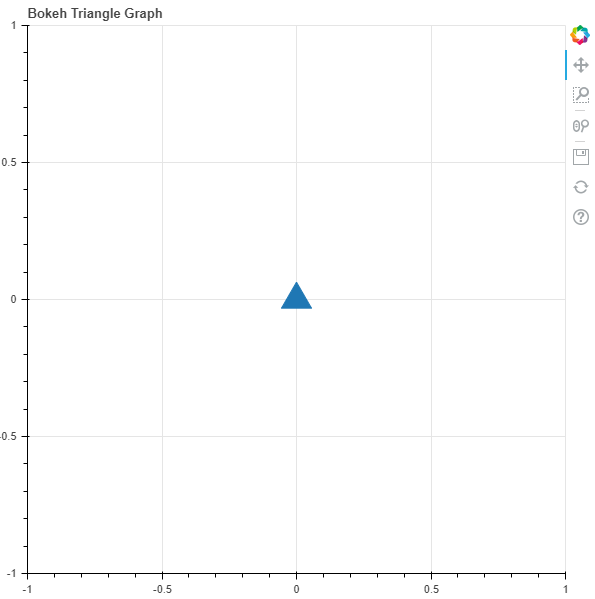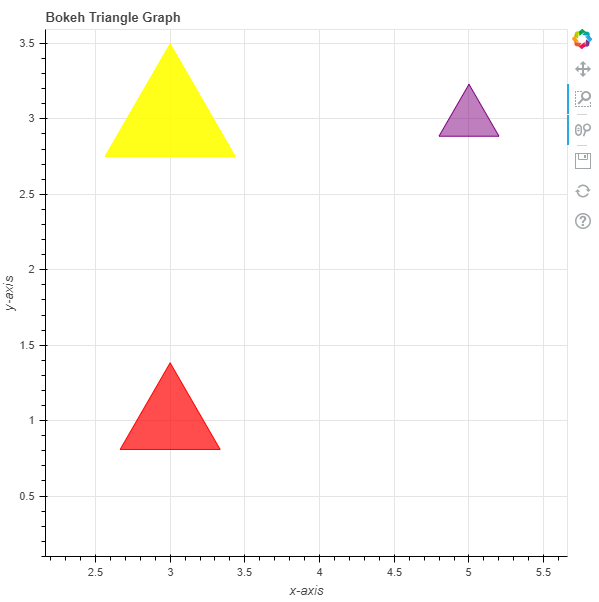# Python Bokeh – Plotting Triangles on a Graph

Bokeh is a Python interactive data visualization. It renders its plots using HTML and JavaScript. It targets modern web browsers for presentation providing elegant, concise construction of novel graphics with high-performance interactivity.

Bokeh can be used to plot triangles on a graph. Plotting triangles on a graph can be done using the `triangle()` method of the `plotting` module.

## plotting.figure.triangle()

Syntax : triangle(parameters)

Parameters :

• x : x-coordinates of the center of the triangle markers
• y : y-coordinates of the center of the triangle markers

Returns : an object of class `GlyphRenderer`

Example 1 : In this example we will be using the default values for plotting the graph.

 `# importing the modules ` `from` `bokeh.plotting ``import` `figure, output_file, show ` `        `  `# file to save the model ` `output_file(``"gfg.html"``) ` `        `  `# instantiating the figure object ` `graph ``=` `figure(title ``=` `"Bokeh Triangle Graph"``) ` `      `  `# the points to be plotted ` `x ``=` `0` `y ``=` `0` `     `  `# plotting the graph ` `graph.triangle(x, y, size ``=` `30``) ` `      `  `# displaying the model ` `show(graph)  `

Output :Example 2 : In this example we will be plotting multiple triangles with various other parameters.

 `# importing the modules  ` `from` `bokeh.plotting ``import` `figure, output_file, show  ` `  `  `# file to save the model  ` `output_file(``"gfg.html"``)  ` `       `  `# instantiating the figure object  ` `graph ``=` `figure(title ``=` `"Bokeh Triangle Graph"``)  ` `  `  `# name of the x-axis  ` `graph.xaxis.axis_label ``=` `"x-axis"` `       `  `# name of the y-axis  ` `graph.yaxis.axis_label ``=` `"y-axis"` `  `  `# points to be plotted ` `x ``=` `[``3``, ``3``, ``5``] ` `y ``=` `[``3``, ``1``, ``3``] ` `size ``=` `[``130``, ``100``, ``60``] ` `  `  `# color value of the triangles ` `color ``=` `[``"yellow"``, ``"red"``, ``"purple"``] ` `  `  `# fill alpha value of the triangles ` `fill_alpha ``=` `[``0.9``, ``0.7``, ``0.5``] ` `  `  `# plotting the graph  ` `graph.triangle(x, y, ` `               ``size ``=` `size, ` `               ``color ``=` `color, ` `               ``fill_alpha ``=` `fill_alpha)  ` `       `  `# displaying the model  ` `show(graph) `

Output :Whether you're preparing for your first job interview or aiming to upskill in this ever-evolving tech landscape, GeeksforGeeks Courses are your key to success. We provide top-quality content at affordable prices, all geared towards accelerating your growth in a time-bound manner. Join the millions we've already empowered, and we're here to do the same for you. Don't miss out - check it out now!

Previous
Next